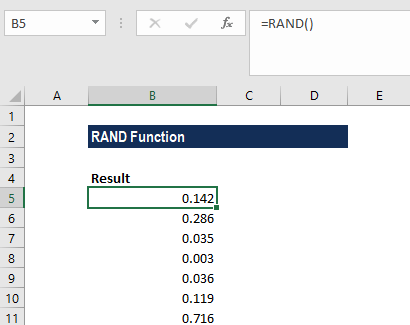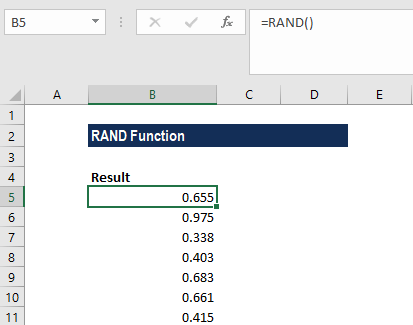# RAND Function

Returns a random number between 0 and 1

## What is the RAND Function?

The RAND function is categorized under Excel Math and Trigonometry functions. The function will return a random number between 0 and 1. It provides a real number that will be returned every time the worksheet is opened.

As a financial analyst, the RAND function can be used to generate random numbers. However, it is used less frequently in the finance industry, as compared to other fields such as cryptography and statistics.

### Formula

=RAND()

The RAND function requires no argument.

### How to use the RAND Function in Excel?

To understand the uses of the RAND function, let’s consider an example:

#### Example 1

When we type =RAND() in any cell, every time the worksheet is opened or recalculated, it will show a different number, as shown below:When we did some recalculations, the worksheet changed the results, as shown below:### Things to remember about the RAND Function

1. RAND will calculate a new value each time the worksheet is calculated. If we wish to stop random numbers from being generated, we need to copy the cells that contain RAND to the clipboard and then use Paste Special > Values to convert them to text.
2. To get a random number that will not change when the worksheet is calculated, we need to enter =RAND() in the Formula bar and then press F9 to convert the formula into its result.
3. If we wish to generate a set of random numbers in multiple cells, we need to select the cells, enter RAND() and then press Ctrl + Enter.
4. If we wish to generate a random number between two numbers, we can use the formula: RAND() * (b – a) + a, where is the smallest number and is the largest number that we wish to generate a random number for.

Thanks for reading CFI’s guide to important Excel functions! By taking the time to learn and master these Excel functions, you’ll significantly speed up your financial analysis. To learn more, check out these additional CFI resources:

• Excel Functions for Finance